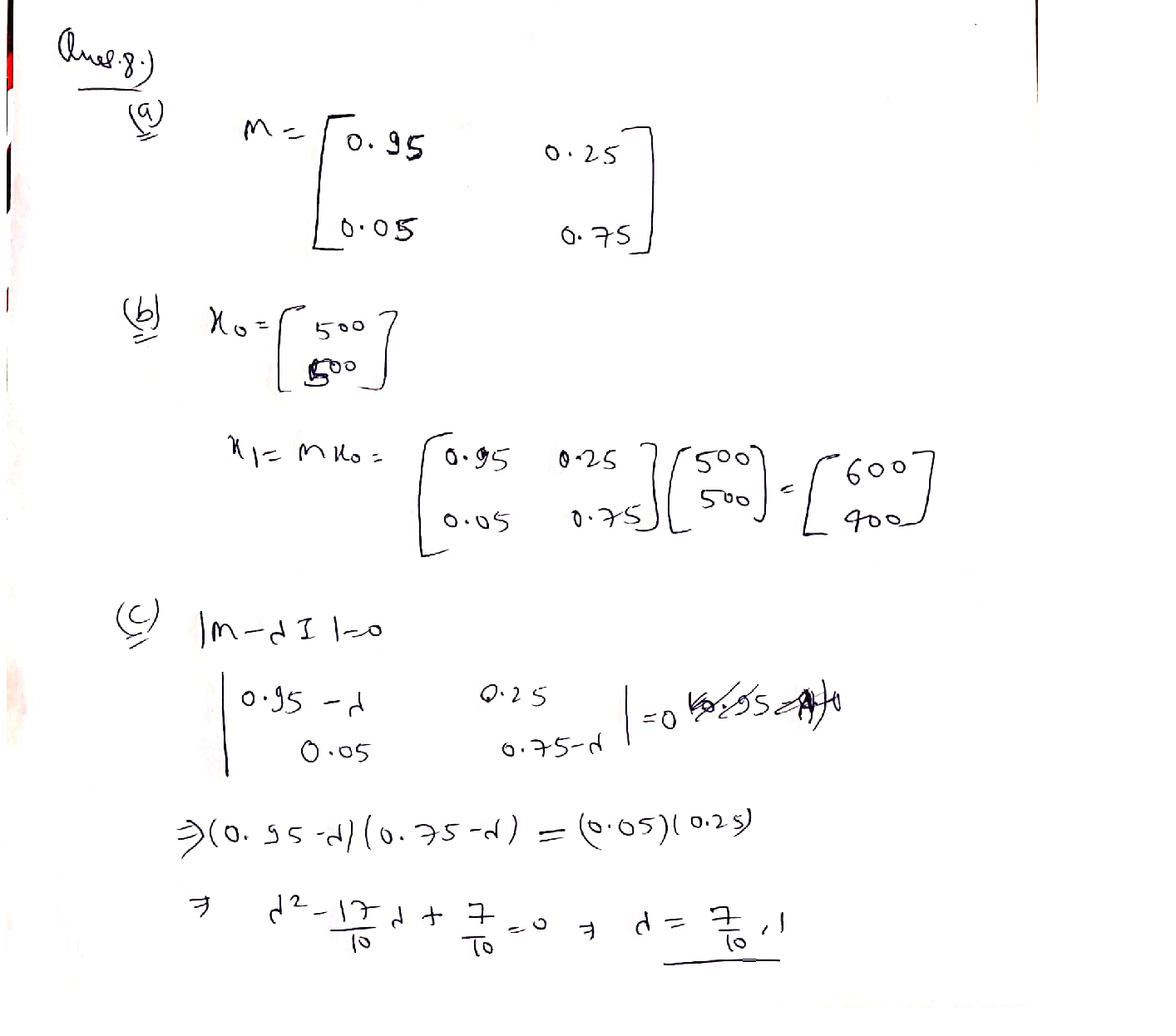Question

Linear Algebra

8. Suppose that, in any given month, the probability that an unemployed person will find employment is 0.25, while the probability that they remain unemployed is 0.75. On the other hand, each month the probability that an employed person will lose their job is 0.05, while the probability that they remain employed is 0.95. The number of people in the labor force is fixed at 1000 (ut + et = 1000 at all times t).

\text { a. Let the state vector at time } t \text { be defined as } x_{t} \equiv\left[\begin{array}{l} e_{t} \\ u_{t} \end{array}\right], \text { where u denotes the number of }

people in the labor force who are unemployed and e denotes the number employed. What

\text { is the Markov transition matrix, } \mathrm{M}, \text { for which } x_{t+1}=M x_{t} ?

b. If half of the labor force is unemployed at time 0, how many people will be employed at time 1?

c. Write down the characteristic equation for M, and use it to find both of M's eigenvalues.

d. What do the eigenvalues tell you about the steady state for this process?

e. What is the steady-state vector, x*, for this process?Verified### Question 45665Linear Algebra

\text { 4] Find } g(0)-g(9)+g(2) \text {, if }
g(x)=\left\{\begin{aligned} \frac{x+1}{2}, & \text { if } x \text { is odd } \\ \frac{x}{2}, & \text { if } x \text { is even } \end{aligned}\right.

### Question 45664Linear Algebra

\text { 3] Find } f(4)-f(2)+f(3) \text {, if }
f(x)=\left\{\begin{array}{ll} \frac{x+1}{2}, & \text { if } x \text { is odd } \\ \frac{x}{4}, & \text { if } x \text { is even } \end{array}\right.

### Question 45663Linear Algebra

2] Find the Domains of the following functions:
\text { a) } f(x)=\sqrt{15-5 x}
f(x)=\frac{x^{2}-2 x+1}{x^{2}-4 x-21}
f(x)=\frac{x^{2}-2 x+1}{\sqrt{16-2 x}}

### Question 45662Linear Algebra

a) Give the definition of a rational function. [5 pts]
b) Give an example of a polynomial function of degree 3. [5 pts]
c) Can a constant function be a polynomial and a rational function at the same time? Explain your answer. [5 pts]
d) Give an example of a non-polynomial function and explain why not apolynomial function. [10 pts)

### Question 45333Linear Algebra

5) Ве,
\begin{array}{c} f: \mathbb{R} \rightarrow \mathbb{R} \\ f(x)=\left\{\begin{array}{ll} x^{2}-3 \cos (\pi x) & x<0 \\ x-4 e^{-2 x} & x \geq 0 . \end{array}\right. \end{array}
Calculate
\int_{-1}^{2} f(x) d x
Presenting the result in simplified form

### Question 45332Linear Algebra

4) Be f: R - Ra function differentiable in R such your derivative f', has in maximum a real zero.
Prove that the equation f(x)=0 has in maximum 2 real square

### Question 45331Linear Algebra

3) Take in consideration the following function
f(x)=\left\{\begin{array}{ll} f: \mathbb{R} \rightarrow \mathbb{R} & \\ \frac{x^{2}-4 x+\cos (\sin (x)),}{x^{4}+4 x^{2}+1}, & x \leq 0 \end{array}\right.
a) Show that the function f is continuous in R+ and in R- but discontinuous in the point X
b) Say justifying, if f is differenciable in X=0

### Question 45330Linear Algebra

2) Prove by definition that
\lim _{x \rightarrow 0} x^{2} \cos \left(e^{x}\right)=0

### Question 45329Linear Algebra

1) Calculate the following limit:
\lim _{x \rightarrow+\infty} \frac{x^{2}\left(e^{-3 x}+1\right)+x \cos (5 x)}{x^{2}+7 x+1}

### Question 45305Linear Algebra

7. Consider the following problem.
Maximize Z = 6X1 + 8X2
5X1 + 2X2 <=20
X1 + 2X2 <=10
X1, X2 >= 0
a. Construct the dual problem for this primal problem
b. Solve both the primal problem and the dual problem graphically.Identify the CPF (Corner-Point Feasible) solutions and corner -pointinfeasible solutions for both problems. Calculate the objectivefunction values for all these solutions.
c. Construct a table listing the complementary basic solutions for theseproblems.
d. Work through the simplex method step by step to solve the primal problem. After each iteration, identify the basic feasible (BF) solution for this problem and the complementary basic solution for the dual problem. Also identify the corresponding corner-point solutions.

### Submit query